# Python – Performing operations on the stock data

• Last Updated : 02 Sep, 2020

This article demonstrates basic operations that can be done using Python to analyze and construct algorithmic trading strategies on stock data. We run through some simple operations that can be performed using Python on stock data, and we begin by reading stock data from a CSV file. Python has emerged as the fastest-growing programming language and this stems from multiple factors such as ease of learning, readability, conciseness, a large group of developers, application across domains, etc. Python has also found broad adoption in trading and this has led to the growth of Python-based analytics tools, Python APIs, and trading strategies using Python.
This post aims to illustrate how easy it is to learn and apply Python to formulate and analyze trading strategies. If you’re new to programming this blog you may just be helping to conquer your programming anxiety. Python comes with the built-in DateTime module to handle the dates and times. It comes with different functions to control the dates and times, as you probably guessed. We can easily parse any date-time string using this module, and convert it to a DateTime object. Let’s run through some simple operations which can be done using Python on stock info. We begin by reading the data from a CSV file on the stock. The CSV file contains the stock numbers Open-High-Low-Close (OHLC) and Volume.

Code:

Attention geek! Strengthen your foundations with the Python Programming Foundation Course and learn the basics.

To begin with, your interview preparations Enhance your Data Structures concepts with the Python DS Course. And to begin with your Machine Learning Journey, join the Machine Learning - Basic Level Course

 `import` `pandas as pd`` ` `# Load data from csv file``data ``=` `pd.read_csv(``'YourFileName.csv'``, index_col ``=``"Date"``, parse_dates ``=` `True``)`` ` `data.head()`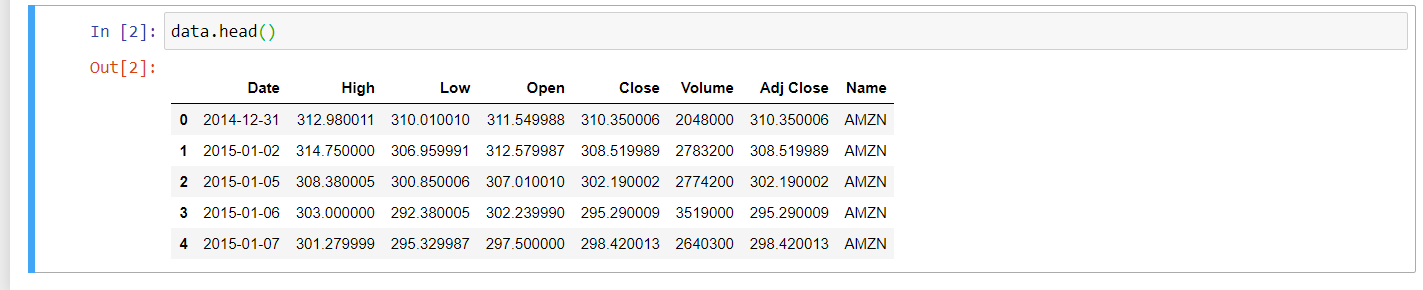Parsing allows us to access data by just mentioning the date or month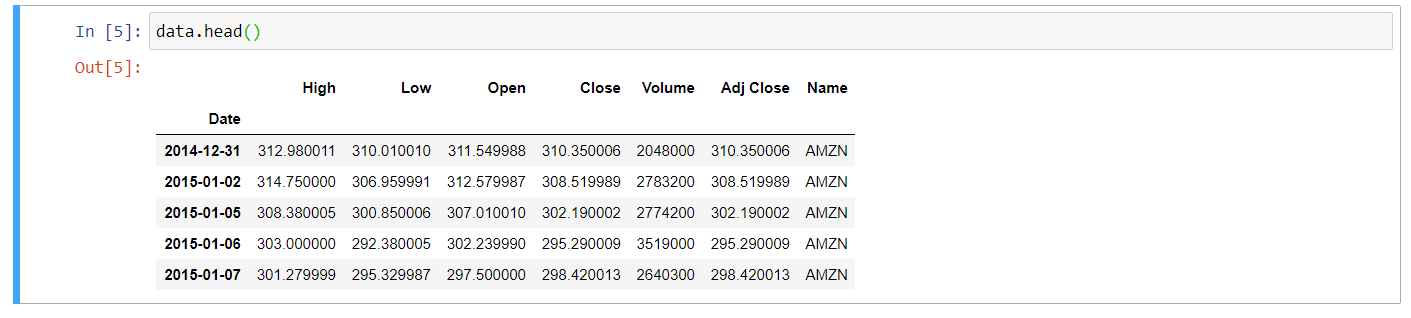Dataframe after parsing date

Determine if the object is a DateTime index for pandas using type()function.

Code:

 `type``(data.index)`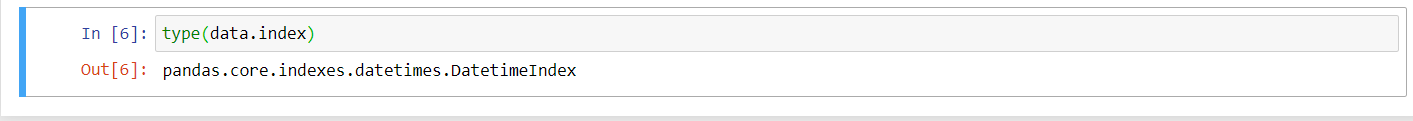Dataframe is a DateTime index

If you want to know the number of trading days (number of rows) in the given set of data,  it can be done using the count() method.

Code:

 `# Number of rows in the data set. You can use any column for that purpose.``# I have used 'Close'``data[``'Close'``].count()`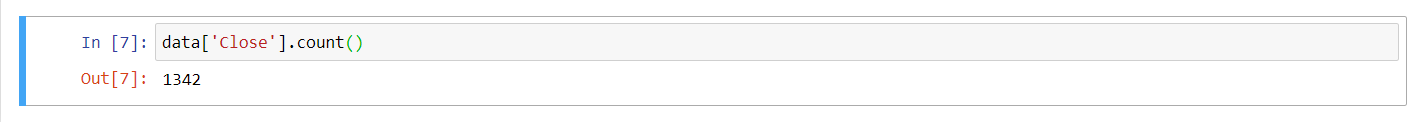No. of rows in a Dataframe.

What is the highest close price achieved over the given period? This is made possible by the use of the max() method.

Code:

 `maximum_price ``=` `data[``'Close'``].``max``()``print``(maximum_price)`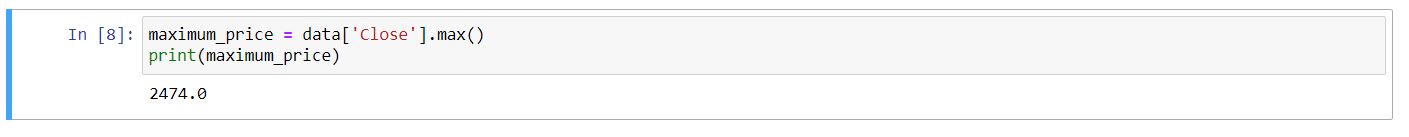Maximum Price

Is it possible to know the date this maximum price was reached on as well? We apply index property as shown below to find the respective date.

Code:

 `data.Close[data.Close ``=``=` `maximum_price].index`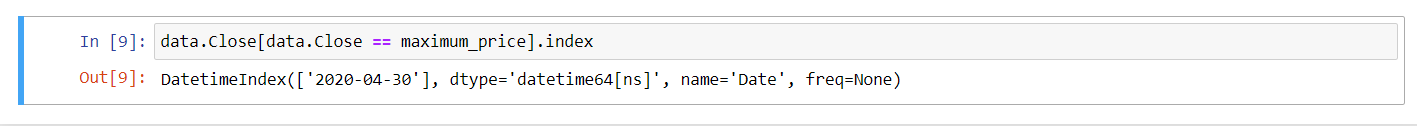The maximum price of the stock was crossed on 30th April 2020

Let’s calculate the change in percentage in closing price per day. We ‘re adding a new ‘Daily_Change’ column to our existing data set. We use pct_change() method to calculate daily returns.

Code:

 `# Compute the percentage change``data[``'Daily_Change'``] ``=` `data[``'Close'``].pct_change()``*``100``data.head()`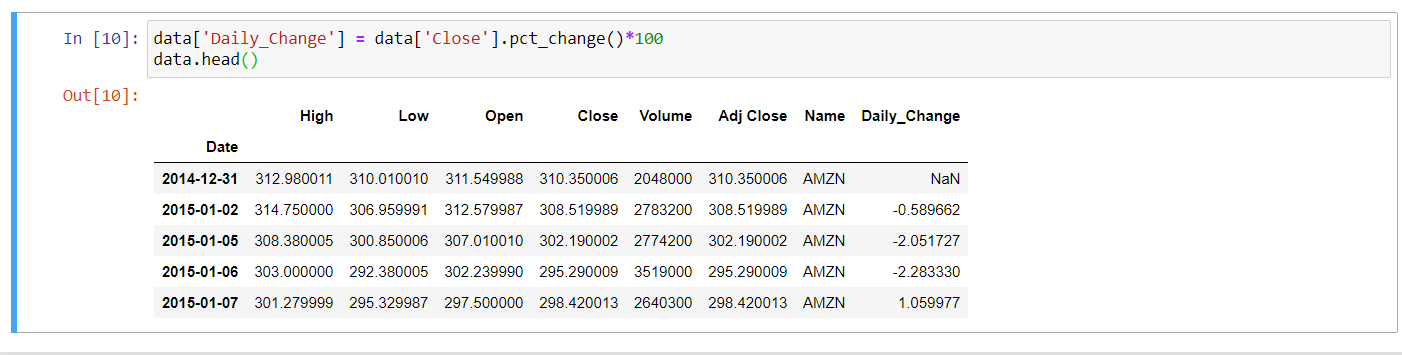Daily returns of the stock

In the end, let’s add a few indicators. We measure the simple moving average of 15 days, and the average volume of 10 days. We will add more measures to our data frame and then evaluate the trend in stocks to see whether it is bullish or bearish.

Code:

 `# Computing mean for 15 days, also known as SMA (Short term moving average)``days ``=` `15``data[``'SMA'``] ``=` `data[``'Close'``].rolling(window ``=` `days).mean()`` ` `# Computing average volume for period of 10 days``data[``'Avg_vol'``] ``=``(data[``'Volume'``]).rolling(window ``=` `10``).mean()``data.tail(``10``)`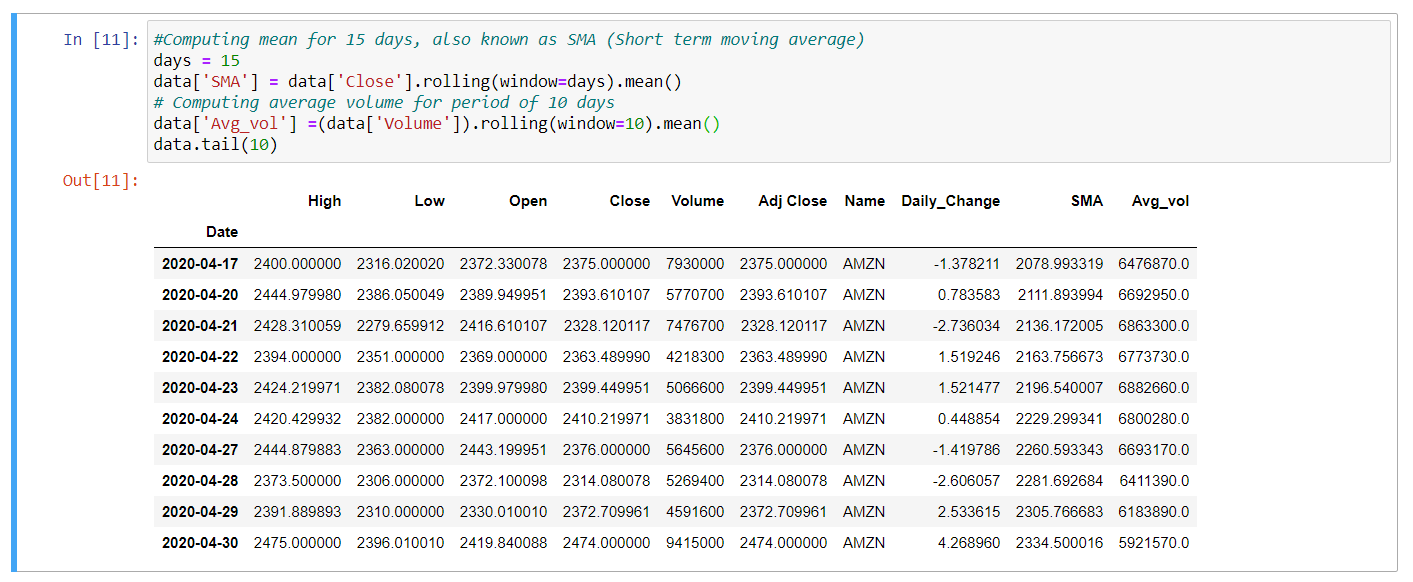We covered a few easy ways in this short post to evaluate the data set and develop greater understanding of stock data. Can you consider constructing a trade strategy using specific basic operations and simple indicators? Simple trading strategies can be efficient and this can be assured by many active traders.

My Personal Notes arrow_drop_up